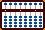The Bead Unbaffled - An Abacus Manual

###Multiplying & Dividing Negative Numbers  - by Shane Baggs

Shane Baggs shares his method in a message to the Yahoo soroban/abacus newsgroup.

#2 is a good point. You solved it by adding 9's, I solved it by working with the "owed" rod. There's more than one way to do things on an abacus!

After multiplying a positive and a negative, subtract the positive from the "owed" (usually 1000's) column of the product.

Before dividing a negative by a positive, add one less than the positive to the "owed" (usually 1000's) column. If there's a remainder in the final answer, convert the positive remainder to negative by adding 1 to the quotient and subtracting the divisor from the remainder.

Until I read your post, I didn't think you could multiply a negative by a negative and get a positive! Here's how I'd handle that:

After multiplying a negative and a negative, subtract each number from the others' "owed" column. Read what's left as a positive number.

September, 2007